# Capacitor AC Response

 Impedance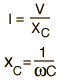CalculateExamine Inductor ResistorContribution tocomplex impedance Phasor diagram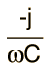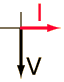You know that the voltage across a capacitor lags the current because the current must flow to build up the charge, and the voltage is proportional to that charge which is built up on the capacitor plates.

Index

Capacitance concepts

Inductance concepts

 HyperPhysics***** Electricity and Magnetism R Nave
Go Back

# Capacitive Reactance

The frequency dependent impedance of a capacitor is called capacitive reactance.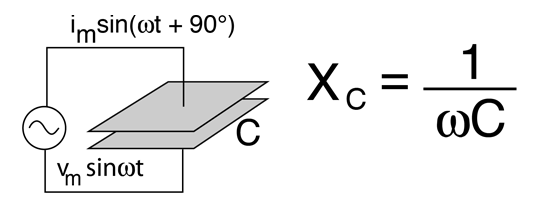This calculation works by clicking on the desired quantity in the expression below. Enter the necessary data and then click on the quantity you wish to calculate. Default values will be entered for unspecified quantities, but all quantities may be changed.

Impedance = 1/(angular frequency)x(capacitance)

 For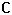= x10^ F =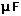= pF
 at angular frequency ω = x10^ rad/s
and frequency = x10^ Hz = kHz = MHz

the capacitive reactance is

 XC = x10^ ohms = kohms = Megohms
 AC behavior of capacitor
Index

Capacitance concepts

Inductance concepts

 HyperPhysics***** Electricity and Magnetism R Nave
Go Back